# Division of Fractions and Whole Numbers

You may find our Fractions - Parts of Numbers page useful to read in conjunction with this article.

## Division of Fractions

Division is the most challenging of the four mathematical operations, right? Thankfully, not when it comes to dealing with fractions.

The key to knowing how to divide fractions is understanding that the operation of multiplying is the opposite of division. If we can make a multiplication sum then it's not too hard - as shown in the previous walkthrough.

To turn a division sum into a multiplication sum, we need to take the fraction which we are dividing by and turn it on its head. Once you've done this (effectively making it the 'opposite' of the number you started with) then you can multiply instead of dividing, and get the same answer!

Let's explore an example: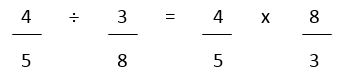By turning the number we're dividing by upside down, while turning the division sign into a multiplication sign, we're keeping the answer the same. Effectively, we're finding the 'opposite of the opposite', which is the same as we started with.

If we carry out the simple multiplication sums, we get: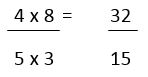Now we have an improper fraction; the way we make it into a mixed number (the regular way of expressing an answer) is to see how many times the bottom number goes into the top. The answer is 2, with a remainder of 2. The answer is therefore: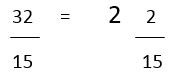Don't be afraid of getting numbers which are larger than the ones you start with. If both numbers are normal fractions then you will get a larger answer than the initial figures.

## Division of Fractions and Whole Numbers

Dividing fractions is not as intimidating as it appears - see the walkthrough - but when you get questions which involve a whole or mixed number as well it may seem harder. In fact, there's only an extra step to take before carrying out the same operations as before. If the mixed number or whole number is turned into a fraction, then you can treat it as a division sum as per the previous example.

Let's see how it works:

What is a half divided by two and a quarter?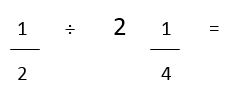The theory says that if you turn the second fraction upside-down then you can simply multiply the digits and get an answer. The problem is that our second figure is not a fraction but it can become one easily enough. With reference to the mixed numbers walkthrough, we need to change the two and a quarter into just quarters. There are four quarters in a whole one, so two and a quarter is the same as nine quarters.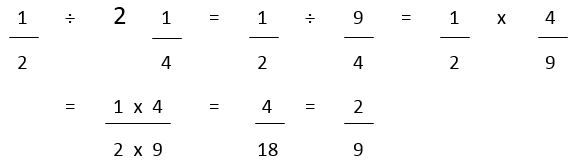The process is just following what we've done before - change the mixed number and then change the division sum to a multiplication. The final step is to simplify the fraction and put it in its lowest form.

What do you do if you encounter a number which is whole, and doesn't have a denominator? Well, 4 is the same as 4 ÷ 1 so can be expressed as 4 over 1. Any number can be expressed as itself over the number one. Do this and you have a fraction with which you can work.

We use cookies to make your experience of our website better.

To comply with the new e-Privacy directive, we need to ask for your consent -